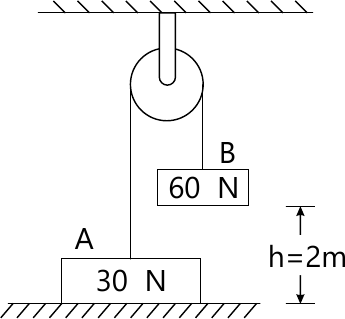# Engineering Mechanics Question paper May 2015

Engineering Mechanics Question paper May 2015 – The engineering mechanics question paper for may 2015 is hard to crack for semester 1 Mumbai engineering students of F.E. first year.

### Engineering Mechanics Question paper May 2015

1 (a) The guy cables AB & AC are attached to the top of the transmission tower as shown in figure 1. The tension in cable AC is 8 KN. Determie the required tension T in cable AB such that the net effect of the two cable tension is a downward force at point A. Determine the magnitude R of this downward force.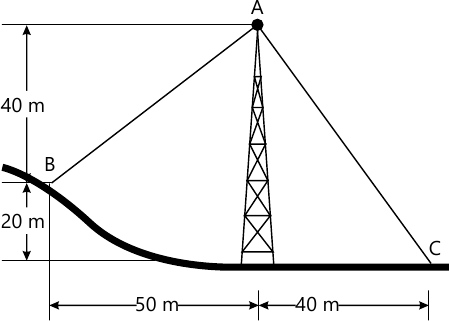4 M
1 (b) Determine the tensions is cords AB & BC for equilibrium of 30 kg block.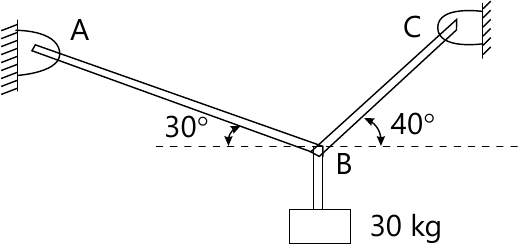4 M
1 (c) A point box weighing 9 kg is kept on a wooden block weighing 1.2 kg (Fig, 3). Determine the magnitude & direction of the friction force exerted by the roof surface on wooden block & normal force exerted by the roof on the wooden block.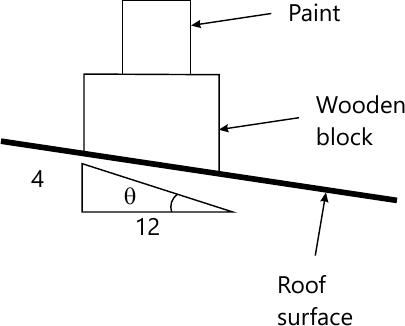4 M
1 (d) Two cars start towards each other from stop X & stop Y at 1:36 PM, the first car reaches stop Y, travelling 8 km path, at 1:44 PM. Second car reaches stop X at 1:46 PM. If they move at uniform velocity, determine their time of meeting & their distance from stop X.
4 M
1 (e) The 550 N box (fig 4) rsts on a horizontal plane for which the coefficient of kinetic friction ?k=0.32. If the box is subjected to a 400N towing force as shown, find the velocity of the box in 4 seconds starting from the rest.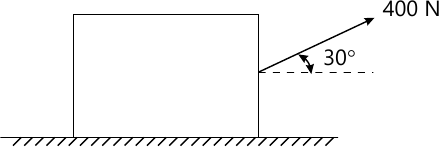4 M
2 (a) Replace the force system (fig 5) by a single force w.r.t to pint C.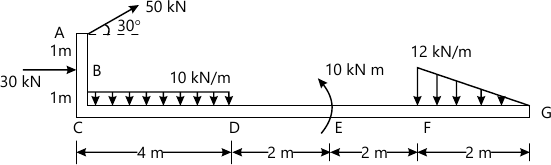6 M
2 (b) A bar of 3m. Length & negligible weight rests in horizontal position on two sooth inclined planes (Fig. 6). Determine the distance x at which the load Q=150 N should be placed from point B to keep the bar horizontal.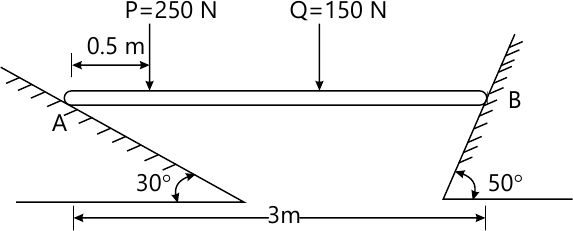8 M
2 (c) Define the terms with neat sketches: Direct impact, oblique impact & line of impact.
6 M
3 (a) Locate the centroid of the shaded portion w.r.t to ox & oy axes (fig 7).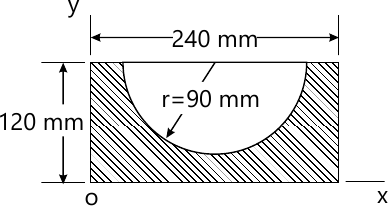8 M
3 (b) A force F =80i +50j-60k passes through a point A(6,2,6). Compute its moment about point B(8,1,4).
6 M
3 (c) The platform P(fig. 8) has negligible mass & is tied down so that the 0.4 m long cords keep a 1 m long spring compressed to 0.6m. When nothing is on the platform. If 4 kg block is placed on the platform & released from rest after the platform is pushed down 0.1 m. Find the maximum height ‘h’ the block rises in the air, measured from the ground. Use Work & Energy Principle.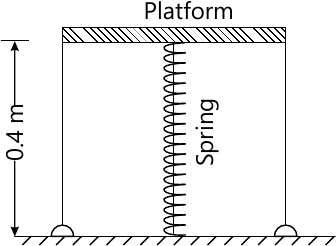6 M
4 (a) Find the support reactions for the beam (Fig 9).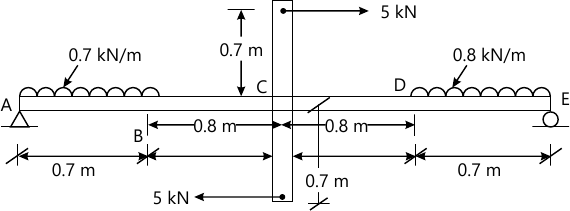8 M
4 (b) Fig. 10 show the v-t diagram for the motion of a train as it moves from station A to station B. Draw a-t graph & find the average speed of the train & the distance between the stations.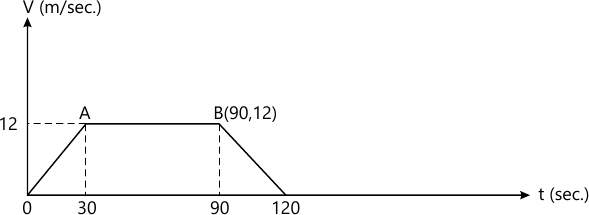6 M
4 (c) A Wheel is attached to the shaft of on electric motor of rated speed of 1740 RPM. When the power is turned on, the unit attains the rated speed in 5 seconds & when the power is turned off, the unit comes to rest in 90 seconds. Assuming uniformly accelerated motion, determine the number of revolutions the unit turns: i) to attain the rated speed & ii) to come to rest.
6 M
5 (a) Using method of joints, find the forces in truss numbers (fig 11).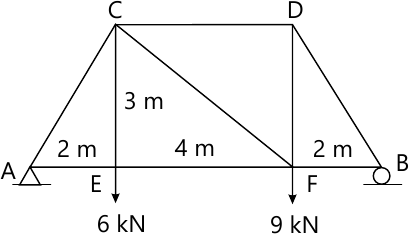8 M
5 (b) The y coordinate of a particle is given by y=6t3-5t. If ax=14t m/sec2 & vx=4 m/sec at t=0, determine the velocity & acceleration of particle when t=1 second.
6 M
5 (c) For crank of concentric mechanism shown in fig 12, determine the instantaneous centre of rotation of connecting rod at position shown. The crank OQ rotates clockwise at 310 RPM. Crank length=10cm, connecting rod length=50 cm. Also find the velocity of P & angular velocity of rod at that instant.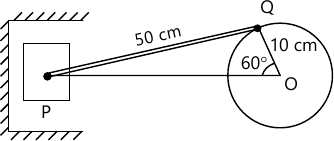6 M
6 (a) Discuss the resultant of concurrent forces in space.
4 M
6 (b) A ladder of 4 m length weighing 200N is placed as shown in fig. 13 ?b=0.25 & ?A=0.35. Calculate the minimum horizontal force to be applied at A to prevent slipping.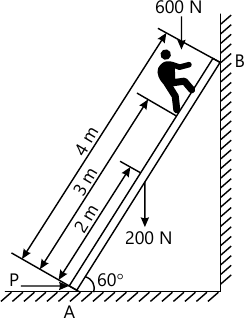8 M
6 (c) With what minimum horizontal velocity (u) can a boy throw a rock at A & have it just clear the obstruction at B? Refer fig. 14.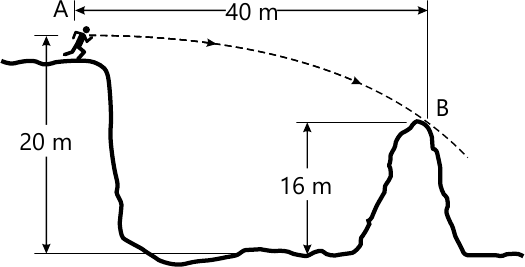4 M
6 (d) Two masses of 60 N, & 30N, are positioned over frictionless & massless pulley (fig. 15). If the 60 N. mass is released from rest, find the speed at which the 60N. mass will hit the ground.